Next: References Up: Computer Vision IT412 Previous: Frequency domain methods

Subsections

# Geometric Transformations

In this section we consider image transformations such as rotation, scaling and distortion (or undistortion!) of images. Such transformations are frequently used as pre-processing steps in applications such as document understanding, where the scanned image may be mis-aligned.

There are two basic steps in geometric transformations:

1.
A spatial transformation of the physical rearrangement of pixels in the image, and
2.
a grey level interpolation, which assigns grey levels to the transformed image

### Spatial transformation

Pixel coordinates (x,y) undergo geometric distortion to produce an image with coordinates (x',y'):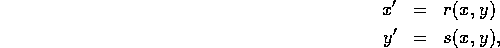where r and s are functions depending on x and y.

Examples:

1.
Suppose,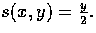This halves the size of the image. This transformation can be represented using a matrix equation2.
Rotation about the origin by an angle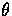is given by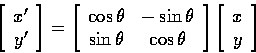Remember the origin of the image is usually the top left hand corner. To rotate about the centre one needs to do a transformation of the origin to the centre of the image first.

### Tie points

Often the spatial transformation needed to correct an image is determined through tie points. These are points in the distorted image for which we know their corrected positions in the final image. Such tie points are often known for satellite images and aerial photos. We will illustrate this concept with the example of correcting a distorted quadrilateral region in an image.

We model such a distortion using a pair of bilinear equations:

x' = c1x + c2y + c3xy + c4

y' = c5x + c6y + c7xy + c8.

We have 4 pairs of tie point coordinates. This enables us to solve for the 8 coefficients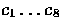.

We can set up the matrix equation using the coordinates of the 4 tie points: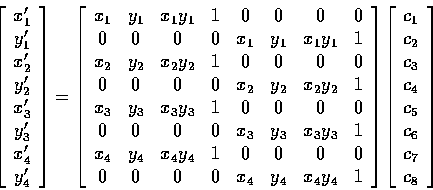In shorthand we can write this equation as

[X'Y'] = [M][C],

which implies

[C] = [M]-1 [X'Y'].

Having solved for the coefficientswe can use them in our original bilinear equations above to obtain the corrected pixel coordinates (x',y') for all pixels (x,y) in the original image within (or near to) the quadrilateral being considered.

To correct for more complex forms of distortion, for example lens distortion, one can use higher order polynomials plus more tie points to generate distortion correction coefficients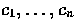.

### Grey Level Interpolation

The problem we have to consider here is that, in general, the distortion correction equations will produce values x' and y' that are not integers. We end up with a set of grey levels for non integer positions in the image. We want to determine what grey levels should be assigned to the integer pixel locations in the output image.

The simplest approach is to assign the grey value for F(x,y) to the pixel having closest integer coordinates to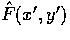. The problem with this is that some pixels may be assigned two grey values, and some may not be assigned a grey level at all - depending on how the integer rounding turns out.

The way to solve this is to look at the problem the other way round. Consider integer pixel locations in the output image and calculate where they must have come from in the input image. That is, work out the inverse image transformation.

These locations in the input image will not (in general) have integer coordinates. However, we do know the grey levels of the 4 surrounding integer pixel positions. All we have to do is interpolate across these known intensities to determine the correct grey level of the position when the output pixel came from.

Various interpolation schemes can be used. A common one is bilinear interpolation, given by

v(x,y) = c1x + c2y + c3xy + c4,

where v(x,y) is the grey value at position (x,y).

Thus we have four coefficients to solve for. We use the known grey values of the 4 pixels surrounding the come from' location to solve for the coefficients.

We need to solve the equation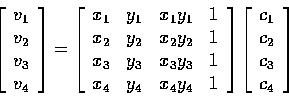or, in short,

[V] = [M][C],

which implies

[C] = [M]-1[V].

This has to be done for every pixel location in the output image and is thus a lot of computation! Alternatively one could simply use the integer pixel position closest to the come from location'. This is adequate for most cases.Next: References Up: Computer Vision IT412 Previous: Frequency domain methods
Robyn Owens
10/29/1997